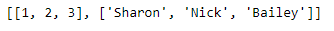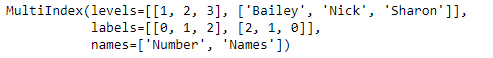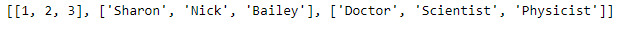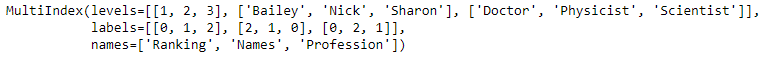# Python | Pandas MultiIndex.from_arrays()

• Last Updated : 24 Dec, 2018

Python is a great language for doing data analysis, primarily because of the fantastic ecosystem of data-centric python packages. Pandas is one of those packages and makes importing and analyzing data much easier.

Pandas` MultiIndex.from_arrays()` function is used to convert arrays into MultiIndex. It is one of the several ways in which we construct a MultiIndex.

Syntax: MultiIndex.from_arrays(arrays, sortorder=None, names=None)

Parameters :
arrays : Each array-like gives one level’s value for each data point. len(arrays) is the number of levels
sortorder : Level of sortedness (must be lexicographically sorted by that level)

Returns: index : MultiIndex

Example #1: Use `MultiIndex.from_arrays()` function to construct a MultiIndex from arrays.

 `# importing pandas as pd``import` `pandas as pd`` ` `# Creating the array``array ``=``[[``1``, ``2``, ``3``], [``'Sharon'``, ``'Nick'``, ``'Bailey'``]]`` ` `# Print the array``print``(array)`

Output :Now let’s create the MultiIndex using this array

 `# Creating the MultiIndex``midx ``=` `pd.MultiIndex.from_arrays(array,``            ``names ``=``(``'Number'``, ``'Names'``))`` ` `# Print the MultiIndex``print``(midx)`

Output :As we can see in the output, the function has created a MultiIndex object using the arrays.

Example #2: Use `MultiIndex.from_arrays()` function to construct a MultiIndex from arrays.

 `# importing pandas as pd``import` `pandas as pd`` ` `# Creating the array``array ``=``[[``1``, ``2``, ``3``], [``'Sharon'``, ``'Nick'``, ``'Bailey'``], ``          ``[``'Doctor'``, ``'Scientist'``, ``'Physicist'``]]`` ` `# Print the array``print``(array)`

Output :Now let’s create the MultiIndex using this array

 `# Creating the MultiIndex``midx ``=` `pd.MultiIndex.from_arrays(array, ``   ``names ``=``(``'Ranking'``, ``'Names'``, ``'Profession'``))`` ` `# Print the MultiIndex``print``(midx)`

Output :As we can see in the output, the function has created a MultiIndex using the passed arrays.

My Personal Notes arrow_drop_up